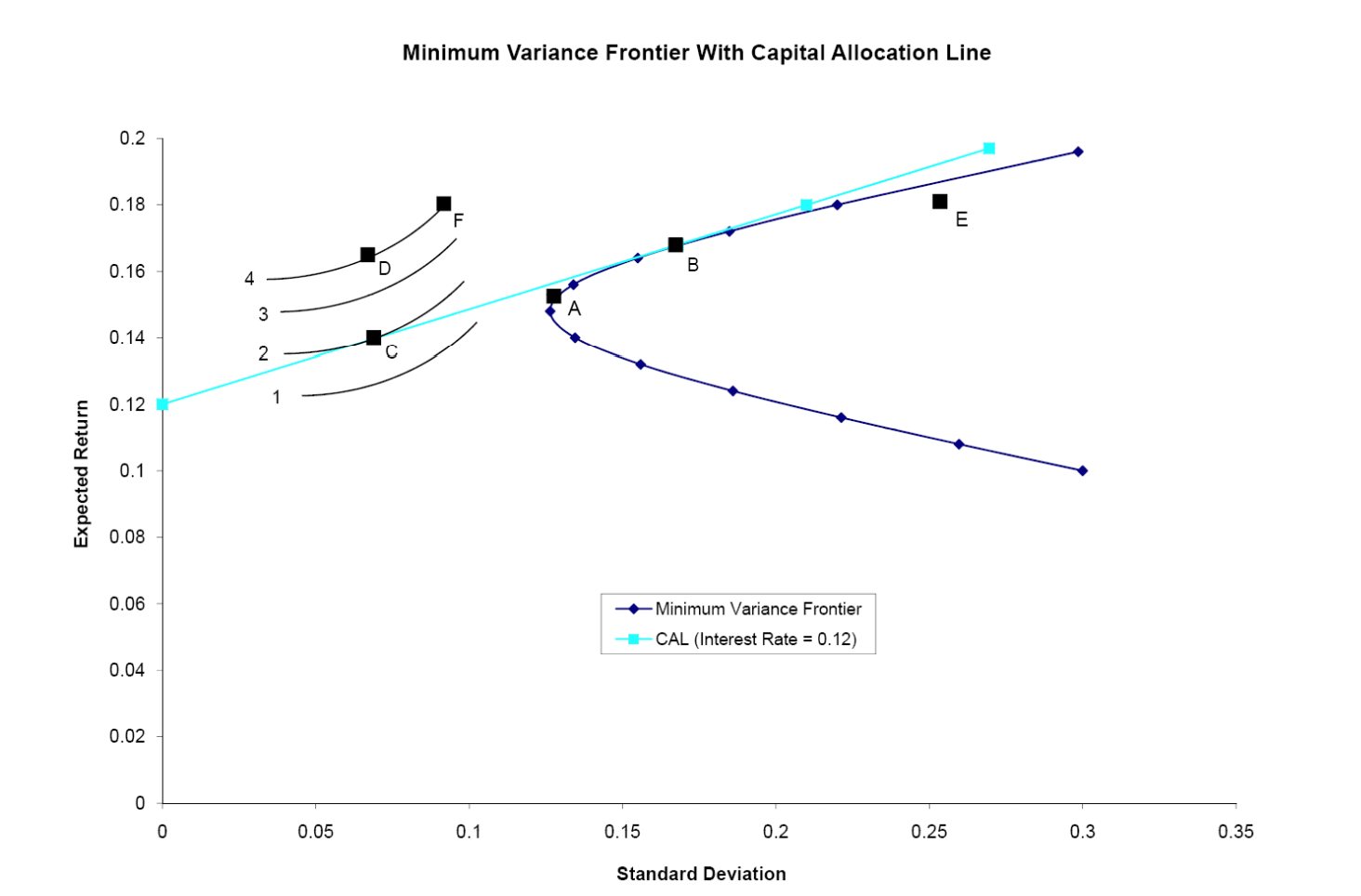### Create an Account

Home / Questions / Use the following graph to answer CFA Problems Which indifference curve represents

# Use the following graph to answer CFA Problems Which indifference curve represents

Use the following graph to answer CFA Problems

Which indifference curve represents the greatest level of utility that can be achieved by the investor?Minimum Variance Frontier With Capital Allocation Line Expected Return Minimum Variance Frontier CAL (Interest Rate = 0.12) 0.05 0.1 0.15 0.2 0.25 0.3 0.35 Standard Deviation

Jan 31 2020 View more View LessGet Solution